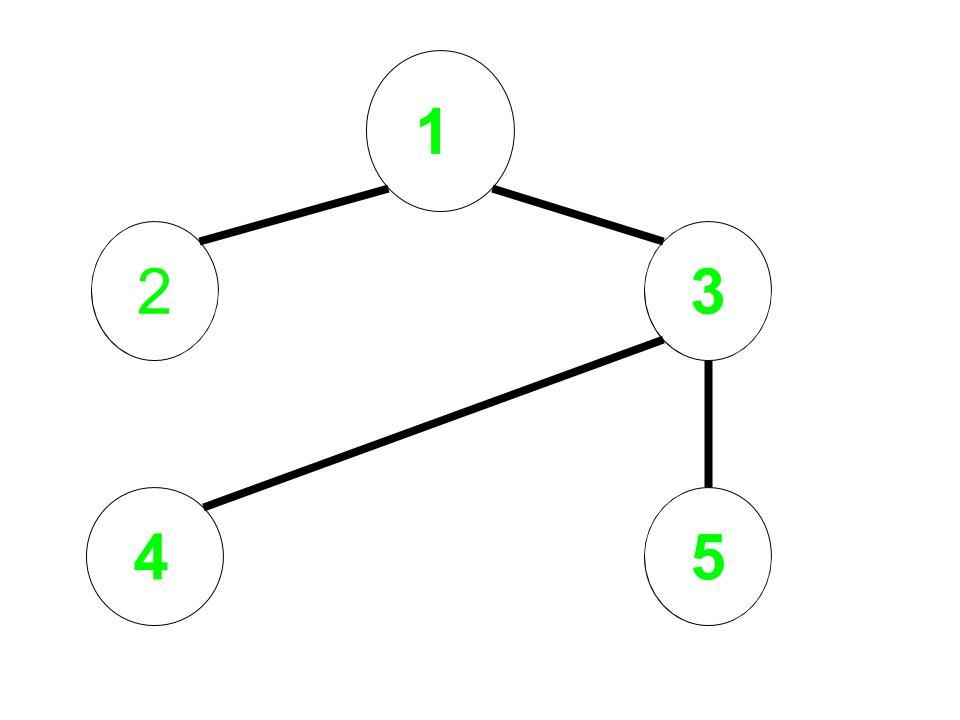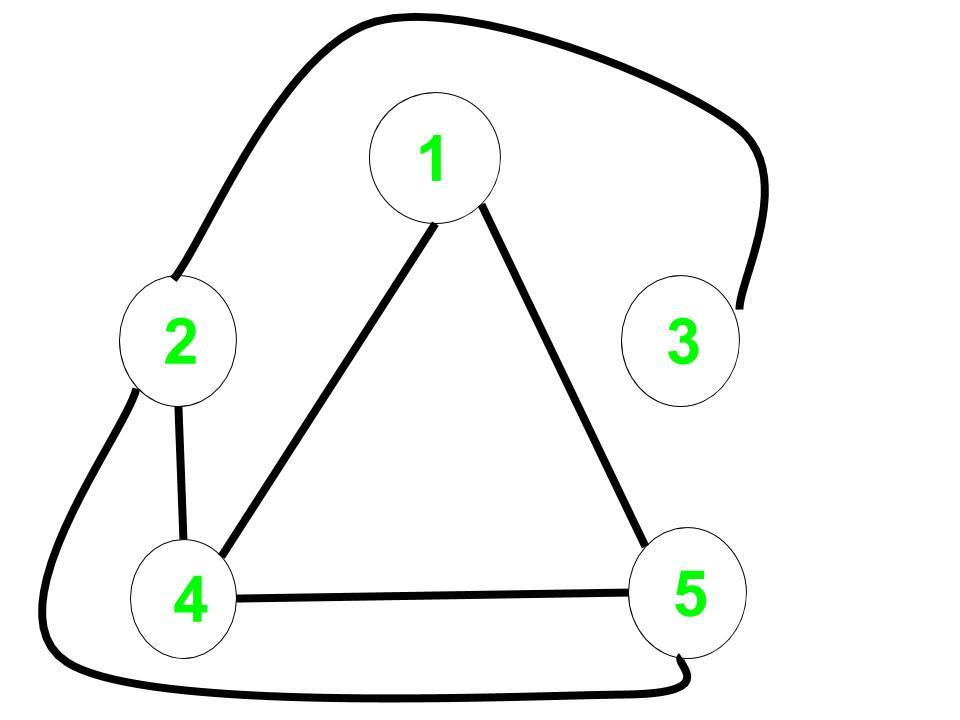# Shortest path in a complement graph

Given an undirected non-weighted graph G. For a given node start return the shortest path that is the number of edges from start to all the nodes in the complement graph of G.

Complement Graph is a graph such that it contains only those edges which are not present in the original graph.

Examples:

Input: Undirected Edges = (1, 2), (1, 3), (3, 4), (3, 5), Start = 1
Output: 0 2 3 1 1
Explanation:
Original Graph:Complement Graph:The distance from 1 to every node in the complement graph are:
1 to 1 = 0,
1 to 2 = 2,
1 to 3 = 3,
1 to 4 = 1,
1 to 5 = 1

## Recommended: Please try your approach on {IDE} first, before moving on to the solution.

Naive Approach: A Simple solution will be to create the complement graph and use Breadth-First Search on this graph to find the distance to all the nodes.

Time complexity: O(n2) for creating the complement graph and O(n + m) for breadth first search.

Efficient Approach: The idea is to use Modified Breadth-First Search to calculate the answer and then there is no need to construct the complement graph.

• For each vertex or node, reduce the distance of a vertex which is a complement to the current vertex and has not been discovered yet.
• For the problem, we have to observe that if the Graph is Sparse then the undiscovered nodes will be visited very fast.

Below is the implementation of the above approach:

 `// CPP implementation to find the ` `// shortest path in a complement graph ` ` `  `#include ` `using` `namespace` `std; ` ` `  `const` `int` `inf = 100000; ` ` `  `void` `bfs(``int` `start, ``int` `n, ``int` `m, ` `         ``map, ``int``> edges) ` `{ ` `    ``int` `i; ` ` `  `    ``// List of undiscovered vertices ` `    ``// initially it will contain all ` `    ``// the vertices of the graph ` `    ``set<``int``> undiscovered; ` ` `  `    ``// Distance will store the distance ` `    ``// of all vertices from start in the ` `    ``// complement graph ` `    ``vector<``int``> distance_node(10000); ` ` `  `    ``for` `(i = 1; i <= n; i++) { ` ` `  `        ``// All vertices are undiscovered ` `        ``undiscovered.insert(i); ` ` `  `        ``// Let initial distance be infinity ` `        ``distance_node[i] = inf; ` `    ``} ` ` `  `    ``undiscovered.erase(start); ` `    ``distance_node[start] = 0; ` `    ``queue<``int``> q; ` ` `  `    ``q.push(start); ` ` `  `    ``// Check if queue is not empty and the ` `    ``// size of undiscovered vertices ` `    ``// is greater than 0 ` `    ``while` `(undiscovered.size() && !q.empty()) { ` `        ``int` `cur = q.front(); ` `        ``q.pop(); ` ` `  `        ``// Vector to store all the complement ` `        ``// vertex to the current vertex ` `        ``// which has not been ` `        ``// discovered or visited yet. ` `        ``vector<``int``> complement_vertex; ` ` `  `        ``for` `(``int` `x : undiscovered) { ` `            ``if` `(edges.count({ cur, x }) == 0) ` `                ``complement_vertex.push_back(x); ` `        ``} ` `        ``for` `(``int` `x : complement_vertex) { ` ` `  `            ``// Check if optimal change ` `            ``// the distance of this ` `            ``// complement vertex ` `            ``if` `(distance_node[x] ` `                ``> distance_node[cur] + 1) { ` `                ``distance_node[x] ` `                    ``= distance_node[cur] + 1; ` `                ``q.push(x); ` `            ``} ` ` `  `            ``// Finally this vertex has been ` `            ``// discovered so erase it from ` `            ``// undiscovered vertices list ` `            ``undiscovered.erase(x); ` `        ``} ` `    ``} ` `    ``// Print the result ` `    ``for` `(``int` `i = 1; i <= n; i++) ` `        ``cout << distance_node[i] << ``" "``; ` `} ` ` `  `// Driver code ` `int` `main() ` `{ ` `    ``// n is the number of vertex ` `    ``// m is the number of edges ` `    ``// start - starting vertex is 1 ` `    ``int` `n = 5, m = 4; ` ` `  `    ``// Using edge hashing makes the ` `    ``// algorithm faster and we can ` `    ``// avoid the use of adjacency ` `    ``// list representation ` `    ``map, ``int``> edges; ` ` `  `    ``// Initial edges for ` `    ``// the original graph ` `    ``edges[{ 1, 3 }] = 1, ` `                 ``edges[{ 3, 1 }] = 1; ` `    ``edges[{ 1, 2 }] = 1, ` `                 ``edges[{ 2, 1 }] = 1; ` `    ``edges[{ 3, 4 }] = 1, ` `                 ``edges[{ 4, 3 }] = 1; ` `    ``edges[{ 3, 5 }] = 1, ` `                 ``edges[{ 5, 3 }] = 1; ` ` `  `    ``bfs(1, n, m, edges); ` ` `  `    ``return` `0; ` `} `

Output:

```0 2 3 1 1
```

Time complexity: O(V+E)
Auxiliary Space: O(V)My Personal Notes arrow_drop_upCheck out this Author's contributed articles.

If you like GeeksforGeeks and would like to contribute, you can also write an article using contribute.geeksforgeeks.org or mail your article to contribute@geeksforgeeks.org. See your article appearing on the GeeksforGeeks main page and help other Geeks.

Please Improve this article if you find anything incorrect by clicking on the "Improve Article" button below.

1

Please write to us at contribute@geeksforgeeks.org to report any issue with the above content.